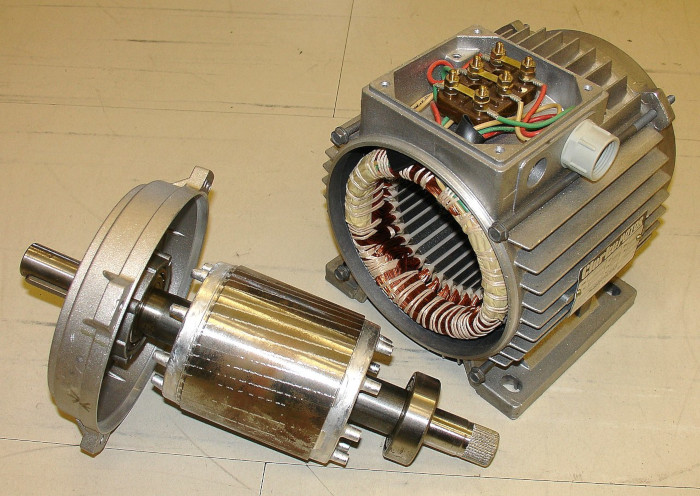# Introduction

When choosing motors and motor controllers for a robotics project the properties of the motor will dictate what needs to be purchased. This Application Note covers the most common motor specifications and what they mean.

# Defining a Few Terms

### Speed

A motor’s speed is the rate at which the shaft rotates. It is usually measure in revolutions per minute (rpm).

### Torque

Torque is a measure of force applied or produced in a rotating system. Larger motors have a torque rating usually measure in foot-pounds and small motors are typically measured in oz-in.

### Current

Current is the measure of the flow of electricity and is measured in amps.

### Power

Power is the amount of work a motor is capable of doing. It is a combination of the speed and torque of a motor, the two multiplied together produces the value of power. Power is measured in watts.Figure 1: Components of typical industrial motor.

# Common Ratings

### Voltage

The voltage or sometime listed as the nominal voltage is the voltage the motor is rated to run at. It is also the voltage at which other values for the motor are rated at. Motors can be run at lower and higher voltages however their performance changes once one deviates from the rated voltage. Higher voltages may extract more performance from the motor but often at the expense of shortening the lifespan of the motor.

This is a measure of the motor’s speed without any load applied to it. The rate a which a loaded motor spins is a function of the amount of load applied. As the load is increased on a motor its speed decreases.

The no-load current is the amount of current the motor draws with no load applied. As with the discussion of no-load speed, the amount of current draw by the motor is a function of the load applied.

### Stall Current

Stall current is the amount of current a motor draws when the shaft is loaded or restricted to the point that it does not rotate. This is the condition under which the motor will draw the maximum amount of current that it can. A stall condition is also likely to do damage to a motor if the condition persists for more than a short length of time. When selecting a motor controller the stall current can be used to select the current rating of the controller since this is the maximum current draw expected. If a motor controller is rated for or above the stall current no damage is likely to the motor controller due to the motor’s current draw.

### Stall Torque

Stall torque is the amount of torque applied to the shaft that will produce zero shaft rotation or the amount of torque the motor can produce at which there is zero shaft rotation. It can be looked at either way, either produced or applied to the motor.

### Rated Current

The rated current of a motor is the maximum current that the motor is rated for. Note that this value is based upon running the motor at the voltage specified for normal operation.

### Rated Power

The rated power of a motor is the maximum power that it is capable of running at. If the rating is specified in watts of kilowatts the values is the electrical power, if the rating is specified in horsepower the rating is for mechanical power. The rated mechanical power of an electric motor is always less that the rated electrical power due to losses in the motor.

### Starting Current

Motors draw large amounts of current when they are first started. The starting current rating is the amount of current that a motor draws when first started and is measured in amps.

# Relationships Between Motor Spec

### Torque vs Speed

There is a linear relationship between torque and speed in an electrical motor. With no load and thus no torque applied the motor will spin at its no-load or maximum speed. As a greater and great load is applied the speed of the motor will decrease up until the stall torque is reached at which point the speed of the shaft will be zero.

### Power vs Torque and Speed

An electric motor will develop it’s maximum power towards the middle of the range of torque and speed. At the extremes of high torque and low speed and low torque and high speed the motor develops the least amount of power.

* Image from Wikimedia Commons. Created by Zureks.